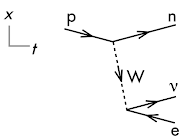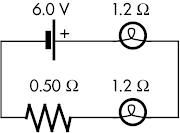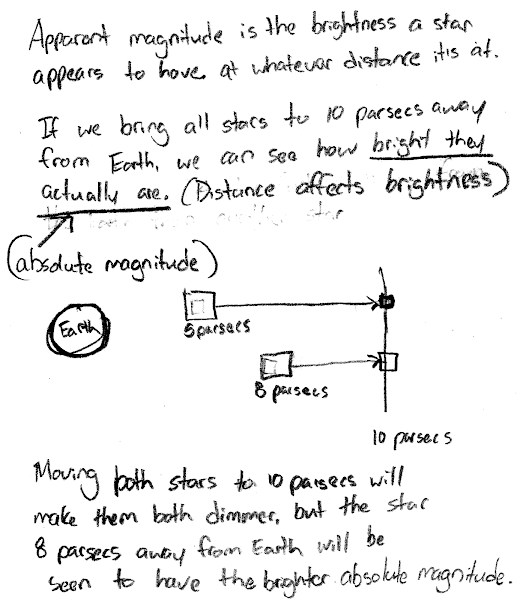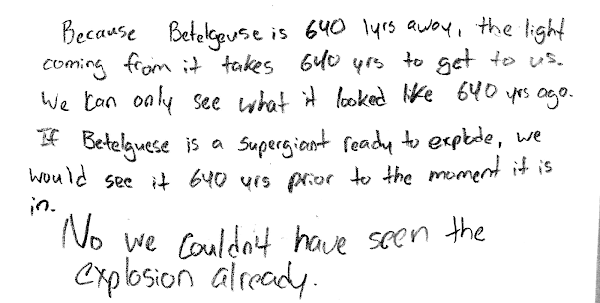20160624

Physics quiz question: thermal contraction of James Webb Space Telescope mirror segment

Physics 205A Quiz 7, fall semester 2015
Cuesta College, San Luis Obispo, CA"Reflections on JWST - May 4, 2016"
James Webb Space Telescope (JWST)
youtu.be/R-ldmlCkAoU

The James Webb Space Telescope will use beryllium mirror segments (linear expansion coefficient 7.0×10–6 K–1), each 1.32 m wide.[*][**] During testing it is cooled from room temperature (293 K) down to its operational temperature of 53 K. The width of a mirror segment will contract by:
(A) 2.2×10–3 m.
(B) 2.7×10–3 m.
(C) 3.2×10–3 m.
(D) 4.2×10–3 m.

[*] P. Hidnert, W. T. Sweeney, "Thermal Expansion of Beryllium and Aluminum Beryllium Alloys," Scientific Papers of the Bureau of Standards, vol. 22 (1927), p. 533, dx.doi.org/10.6028/nbsscipaper.251.
[**] jwst.nasa.gov/mirrors.html.

Correct answer (highlight to unhide): (A)

The relation between the change ∆L of a linear dimension L (here, width) due to a temperature change ∆T is given by:

α·∆T = ∆L/L,

such that:

L = L·α·∆T = (1.32 m)·(7.0×10–6 K–1)·(53 K – 293 K) = –2.2176×10–3 m,

or to two significant figures, the width of the mirror segment decreases by 2.2×10–3 m.

(Response (B) is L·α·(293 K); response (C) is L·α·(53 K + 293 K); response (D) is 1/∆T.)

Sections 70854, 70855, 73320
Exam code: quiz07zSOL
(A) : 62 students
(B) : 5 students
(C) : 1 student
(D) : 1 student

Success level: 90%
Discrimination index (Aubrecht & Aubrecht, 1983): 0.22

Physics quiz question: rate of thermal loss through sleeping pad

Physics 205A Quiz 7, fall semester 2015
Cuesta College, San Luis Obispo, CA"Therm-a-Rest® Closed-Cell Mattresses"
Therm-a-Rest
youtu.be/SwWevyXpibg

A Thermarest® Z Lite SOL™ polyethylene insulating sleeping pad[*]  (area of 0.933 m2 and thickness of 0.020 m) has a thermal resistance of 0.43 K/watt. If there is a 20° C temperature difference between the top (in contact with a sleeping person) and bottom (in contact with the cold ground) of the sleeping pad, the rate of heat per time conducted through the pad will be:
(A) 1.1×10–3 watts.
(B) 2.2×10–2 watts.
(C) 8.6 watts.
(D) 47 watts.

Correct answer (highlight to unhide): (D)

The rate of heat per time conducted is given by:

Power = ∆T/R,

where the temperature difference ∆T and the thermal resistance R of the sleeping pad are given, such that:

Power = (20° C)/(0.43 K/watt) = 46.511627907 watts,

or to two significant figures, the rate of heat conducted through the sleeping pad is 47 watts.

(Response (A) is d/(A·∆T); response (B) is R/∆T; response (C) is R·∆T.)

Sections 70854, 70855, 73320
Exam code: quiz07zSOL
(A) : 3 students
(B) : 4 students
(C) : 8 students
(D) : 54 students

Success level: 78%
Discrimination index (Aubrecht & Aubrecht, 1983): 0.46

20160622

Physics quiz question: comparing surface temperatures of Bellatrix and Pollux

Physics 205A Quiz 7, fall semester 2015
Cuesta College, San Luis Obispo, CA

Stars can be modeled as spherical blackbodies that radiate heat out to an environment assumed to have a temperature of 0 K. Pollux is a star that radiates less heat per time, but is larger in size than Bellatrix. Pollux has a surface temperature __________ Bellatrix.
(A) cooler than.
(B) same as.
(C) hotter than.
(D) (Not enough information given.)

The net power radiated by a star with an environment assumed to be at absolute zero is given by:

Power = –e·σ·A·((T)4 – (Tenv)4) = –e·σ·A·((T)4 – (0 K)4).

where the emissitivity e = 1 (for an ideal blackbody), the Stefan-Boltzmann constant is σ = 5.670×10–8 watts/(m2·K4), A is the surface area, and the surface temperature T is unknown, and can be solved for:

(T)4 = –Power/(e·σ·A),

T = (–Power/(e·σ·A))(1/4).

This allows a ratio to be set up to compare the surface temperatures of Bellatrix and Pollux:

TBellatrix = (–PowerBellatrix/(e·σ·ABellatrix))(1/4),

TPollux = (–PowerPollux/(e·σ·APollux))(1/4),

such that:

(TBellatrix/TPollux) = (PowerBellatrix/PowerPollux))·(APollux/ABellatrix)(1/4).

Since Pollux radiates less heat per time than Bellatrix, then the ratio (PowerBellatrix/PowerPollux)) is greater than 1; and since Pollux is larger in size than Bellatrix, the ratio (APollux/ABellatrix)(1/4) is also greater than 1. Thus the ratio (TBellatrix/TPollux) must then be greater than 1, so Pollux has a cooler surface temperature than Bellatrix.

Sections 70854, 70855
Exam code: quiz07zSOL
(A) : 50 students
(B) : 12 students
(C) : 7 students
(D) : 0 students

Success level: 72%
Discrimination index (Aubrecht & Aubrecht, 1983): 0.70

Physics quiz question: surface temperature of Bellatrix

Physics 205A Quiz 7, fall semester 2015
Cuesta College, San Luis Obispo, CA

Stars can be modeled as spherical blackbodies that radiate heat out to an environment assumed to have a temperature of 0 K. Bellatrix is a star that radiates heat per time at a rate of 2.5×1030 watts, and has a surface area of 2.2×1020 m2. The surface temperature of Bellatrix is:
(A) 4.2×103 K.
(B) 2.1×104 K.
(C) 1.1×105 K.
(D) 6.1×105 K.

The net power radiated by Bellatrix (–2.5×1030 watts, where the negative sign indicates that heat is continuously leaving the surface of the star) given by:

Power = –e·σ·A·((T)4 – (Tenv)4) = –e·σ·A·((T)4 – (0 K)4),

where the emissitivity e = 1 (for an ideal blackbody), the Stefan-Boltzmann constant σ = 5.670×10–8 watts/(m2·K4), A is the surface area, and the surface temperature T is unknown, and can be solved for:

(T)4 = –Power/(e·σ·A),

T = (–Power/(e·σ·A))(1/4),

T = (–(–2.5×1030 watts)/((1)·(5.670×10–8 watts/(m2·K4))·(2.2×1020 m2)))(1/4),

T = 2.11584362479×104 K,

or to two significant figures, the surface temperature of Bellatrix is 2.2×104 K.

(Response (A) is σ(–1/2); response (C) is (Power/A)(–1/2); response (D) is (Power·σ)(1/4).)

Sections 70854, 70855
Exam code: quiz07zSOL
(A) : 12 students
(B) : 31 students
(C) : 15 students
(D) : 10 students

Success level: 45%
Discrimination index (Aubrecht & Aubrecht, 1983): 0.35

20160615

Physics quiz question: electron capture decay of iodine-125

Physics 205B Quiz 7, spring semester 2016
Cuesta College, San Luis Obispo, CA

￼I(125,53) is used as a radiation source placed surgically implanted next to tumors for treatment of eye cancer[*]. ￼I(125,53) ￼decays via electron capture (with a half life of 59.43 days) to become:
(A) Te(123, 52)￼.
(B) Te(125, 52)￼.
(C) Xe(125, 52)￼.
(D) Xe(127, 52)￼.

Correct answer (highlight to unhide): (B)

Electron capture takes in an electron to covert a proton into a neutron, which also leaves the nucleon number the same (a total of 125 protons and neutrons), but decreases the atomic number by one (from 53 protons to 52 protons).

(Response (C) corresponds to ￼the daughter product of I(125,53) undergoing β decay.)

Sections 30882, 30883
Exam code: quiz07eYE5
(A) : 1 student
(B) : 32 students
(C) : 7 students
(D) : 0 students

Success level: 80%
Discrimination index (Aubrecht & Aubrecht, 1983): 0.13

Physics quiz question: interpreting β+ decay Feynman diagram

Physics 205B Quiz 7, spring semester 2016
Cuesta College, San Luis Obispo, CAThis (valid) Feynman diagram depicts the __________ process.
(A) α.
(B) β￼.
(C) β+￼.
(D) electron capture.
(E) γ.

Correct answer (highlight to unhide): (C)

This diagram shows β+ decay, where a proton transforms into a neutron, and the intermediate W+ particle decays into a neutrino and a positron, as shown by the left-to-right neutrino arrow, and a right-to-left electron arrow (corresponding to a positron--the "β+￼" particle--progressing left-to-right as time progresses).

Sections 30882, 30883
Exam code: quiz07eYE5
(A) : 2 students
(B) : 3 students
(C) : 30 students
(D) : 5 students
(E) : 0 students

Success level: 75%
Discrimination index (Aubrecht & Aubrecht, 1983): 0.60

20160614

Physics quiz question: ammeter reading, connected in series with voltmeter

Physics 205B Quiz 5, spring semester 2016
Cuesta College, San Luis Obispo, CAAn ideal 3.2 V emf source is connected to an ideal voltmeter and ammeter, a light bulb, and a resistor. The light bulb is lit. The ammeter reading is:
(A) zero.
(B) some finite, non-zero value below 0.67 A.
(C) exactly 0.67 A.
(D) some finite value above 0.67 A.
(E) ∞.

Correct answer (highlight to unhide): (A)

An ideal ammeter has zero resistance, but it is connected in series to an ideal voltmeter, which has an infinite resistance. Thus no current will flow through the upper loop of this circuit, such that the ammeter will read zero.

(Response (C) is the amount of current flowing through the emf, light bulb, and resistor in the lower loop of this circuit.)
Sections 30882, 30883
Exam code: quiz05Tt1p
(A) : 17 students
(B) : 5 students
(C) : 11 students
(D) : 4 students
(E) : 1 student

Success level: 45%
Discrimination index (Aubrecht & Aubrecht, 1983): 0.26

Physics quiz question: power dissipated by light bulb in series circuit

Physics 205B Quiz 5, spring semester 2016
Cuesta College, San Luis Obispo, CAAn ideal 6.0 V emf source is connected to two light bulbs (each with a 1.2 Ω resistance), and a 0.50 Ω resistor. The electrical power used by the top light bulb is:
(A) 2.1 W.
(B) 5.1 W.
(C) 12 W.
(D) 30 W.

Correct answer (highlight to unhide): (B)

The equivalent resistance of this series circuit is the arithmetic sum of their resistances:

Req = 1.2 Ω + 1.2 Ω + 0.50 Ω = 2.9 Ω.

From Kirchhoff's junction rule, the amount of current flowing through the emf source and all three resistive circuit elements must be the same, as they are in series with each other, and the current flowing this circuit is given by applying Ohm's law to the entire equivalent circuit:

Ieq = ε/Req = (6.0 V)/(2.9 Ω).

Since we now know the resistance value of the top light bulb, and the amount of current flowing through it, the power dissipated by the top light bulb is then:

Ptop light bulb = Ieq·ΔVtop light bulb = Ieq·(Ieq·Rtop light bulb) = Ieq2·Rtop light bulb,

Ptop light bulb = ((6.0 V)/(2.9 Ω))2·(1.2 Ω) = 5.1367419737 W,

or to two significant figures, 5.1 W.

(Response (A) is the numerical value for the current flowing through the circuit Ieq = ε/Req = (6.0 V)/(2.9 Ω); response (C) is ((6.0 V)/(2.9 Ω))2·(2.9 Ω), which is the amount of power supplied by the emf source to rest of the circuit; response (D) is (6.0 V)2/(1.2 Ω), which would be the power dissipated by the top light bulb if it were to use up all of the voltage supplied by the emf (which would violate Kirchhoff's loop rule!).)

Sections 30882, 30883
Exam code: quiz05Tt1p
(A) : 4 students
(B) : 16 students
(C) : 13 students
(D) : 5 students

Success level: 43%
Discrimination index (Aubrecht & Aubrecht, 1983): 0.79

20160613

Physics quiz question: step-down transformer output voltage

Physics 205B Quiz 6, spring semester 2016
Cuesta College, San Luis Obispo, CA

A step-up transformer with 20 times more coils in the secondary than in the primary coil is used with an input voltage of 120 V, and an input current of 0.50 A in the primary coil. The current in the secondary coil is:
(A) 2.5×10–2 A.
(B) 6.0 A.
(C) 10 A.
(D) 2.4×103 A.

Correct answer (highlight to unhide): (A)

The transformer equations are:

ε21 = N2/N1 = I1/I2,

where the input (alternating root-mean-square) voltage and current in the primary coil are ε1 = 120 V and I1 = 0.50 A, respectively. Since the secondary coil has 20 times more turns than the primary, then the output voltage ε2 will be stepped up by a factor of 20, while the output current I2 will be stepped down by a factor of 20:

I2 = I1·(N1/N2),

I2 = (0.50 A)·(1/20) = 2.5×10–2 A.

Response (B) is ε1·(N1/N2); response (C) is I1·(N2/N1); response (D) is ε1·(N2/N1).

Sections 30882, 30883
Exam code: quiz06eL3k
(A) : 24 students
(B) : 3 students
(C) : 10 students
(D) : 2 students

Success level: 62%
Discrimination index (Aubrecht & Aubrecht, 1983): 1.00

20160609

Astronomy quiz question: Milky Way's oldest stars

Astronomy 210 Quiz 7, spring semester 2016
Cuesta College, San Luis Obispo, CA

According to the monolithic collapse model, the oldest stars in the Milky Way are located:
(A) in the halo.
(B) in the nuclear bulge.
(C) within the spiral arms.
(D) inside the central supermassive black hole.

Correct answer (highlight to unhide): (A)

According to the monolithic collapse model, the Milky Way evolved from a spherical shape to its current disk shape, leaving behind the globular clusters out in its halo. Thus globular clusters should all have the identical (old) ages (as determined from the lack of metal absorption lines, and their H-R diagram turn-off points), while the nuclear bulge and the spiral arms should be comprised of mixed-age (old and newer) stars.

Section 30674
Exam code: quiz07N4rK
(A) : 10 students
(B) : 1 student
(C) : 6 students
(D) : 0 students

"Success level": 61% (including partial credit for multiple-choice)
Discrimination index (Aubrecht & Aubrecht, 1983): 0.47

20160608

Astronomy final exam question: brighter absolute magnitude star?

Astronomy 210 Final Exam, spring semester 2016
Cuesta College, San Luis Obispo, CA

Two stars have the same apparent magnitude m, but one is 15 parsecs away from Earth, while the other is 18 parsecs away from Earth. Determine which star has a brighter absolute magnitude M, and discuss how you know this. Explain using the relationships between apparent magnitude, absolute magnitude, and distance.

Solution and grading rubric:
• p:
Correct. Understands difference between apparent magnitude m (brightness as seen from Earth, when placed at their actual distance from Earth) and absolute magnitude M (brightness as seen from Earth, when placed at the "comparison distance" of 10 parsecs away), and discusses:
1. relocating a star that is farther than 10 parsecs away to the closer "comparison distance" of 10 parsecs will increase its apparent magnitude to become its absolute magnitude; and
2. because the star located 18 parsecs away will need to travel a greater distance to be brought to the "comparison distance" than the star located 15 parsecs away, this star will experience a greater increase in brightness; such that
3. the star that was located 18 parsecs away will have a brighter absolute magnitude M than the star that was located 15 parsecs away.
• r:
Nearly correct (explanation weak, unclear or only nearly complete); includes extraneous/tangential information; or has minor errors.
• t:
Contains right ideas, but discussion is unclear/incomplete or contains major errors. At least discussion demonstrates understanding of relationships between apparent magnitudes, absolute magnitudes, and distances.
• v:
Limited relevant discussion of supporting evidence of at least some merit, but in an inconsistent or unclear manner. At least attempts to use relationships between apparent magnitudes, absolute magnitudes, and distances.
• x:
Implementation/application of ideas, but credit given for effort rather than merit. Discussion based on garbled definitions of, or not based on proper relationships between apparent magnitudes, absolute magnitudes, and distances.
• y:
Irrelevant discussion/effectively blank.
• z:
Blank.
Section 30676
Exam code: finalSre6
p: 26 students
r: 0 students
t: 8 students
v: 1 student
x: 2 students
y: 4 students
z: 1 student

A sample "p" response (from student 8825):Astronomy final exam question: brighter absolute magnitude star?

Astronomy 210 Final Exam, spring semester 2016
Cuesta College, San Luis Obispo, CA

Two stars have the same apparent magnitude m, but one is 5 parsecs away from Earth, while the other is 8 parsecs away from Earth. Determine which star has a brighter absolute magnitude M, and discuss how you know this. Explain using the relationships between apparent magnitude, absolute magnitude, and distance.

Solution and grading rubric:
• p:
Correct. Understands difference between apparent magnitude m (brightness as seen from Earth, when placed at their actual distance from Earth) and absolute magnitude M (brightness as seen from Earth, when placed at the "comparison distance" of 10 parsecs away), and discusses:
1. relocating a star that is closer than 10 parsecs away to the farther "comparison distance" of 10 parsecs will decrease its apparent magnitude to become its absolute magnitude; and
2. because the star located 5 parsecs away will need to travel a greater distance to be brought to the "comparison distance" than the star located 8 parsecs away, this star will experience a greater increase in brightness; such that
3. the star that was located 5 parsecs away will have a dimmer absolute magnitude M than the star that was located 8 parsecs away.
• r:
Nearly correct (explanation weak, unclear or only nearly complete); includes extraneous/tangential information; or has minor errors.
• t:
Contains right ideas, but discussion is unclear/incomplete or contains major errors. At least discussion demonstrates understanding of relationships between apparent magnitudes, absolute magnitudes, and distances.
• v:
Limited relevant discussion of supporting evidence of at least some merit, but in an inconsistent or unclear manner. At least attempts to use relationships between apparent magnitudes, absolute magnitudes, and distances.
• x:
Implementation/application of ideas, but credit given for effort rather than merit. Discussion based on garbled definitions of, or not based on proper relationships between apparent magnitudes, absolute magnitudes, and distances.
• y:
Irrelevant discussion/effectively blank.
• z:
Blank.
Section 30674
Exam code: finalNKPd
p: 7 students
r: 3 students
t: 2 students
v: 2 students
x: 0 students
y: 0 students
z: 0 students
A sample "p" response (from student 1503):Astronomy final exam question: atoms in our bodies produced by red dwarfs?

Astronomy 210 Final Exam, spring semester 2016
Cuesta College, San Luis Obispo, CA

An astronomy question on an online discussion board[*] was asked:
Is it possible that any of the atoms in our bodies were made by red dwarfs?
Discuss why none of the atoms in our bodies could have been made by red dwarfs. Explain using the properties and evolution of stars.

Solution and grading rubric:
• p:
Correct. Understands that atoms in our bodies were created by a previous generation of massive and medium-mass stars that exploded as type II or type Ia supernovae, which were capable of producing metals and scattering these atoms to be subsequently incorporated into the formation of our solar system and our bodies. Discusses how none of the atoms in our bodies can have been made by red dwarfs using at least one of the following or similar arguments:
1. red dwarfs are incapable of metal formation (undergoing post-hydrogen fusion, or type II or type Ia supernova); or
2. no red dwarfs have ever died yet (and thus they cannot contribute non-metals to us by some unforseen process that would occur in the far future).
• r:
Nearly correct (explanation weak, unclear or only nearly complete); includes extraneous/tangential information; or has minor errors.
• t:
Contains right ideas, but discussion is unclear/incomplete or contains major errors. Typically argues how younger, shorter-lived stars would have inherited their metals from earlier generations of stars undergoing type II or type Ia supernovae.
• v:
Limited relevant discussion of supporting evidence of at least some merit, but in an inconsistent or unclear manner. Garbled discussion of properties and evolution of stars.
• x:
Implementation/application of ideas, but credit given for effort rather than merit. Discussion other than that of the properties and evolution of stars.
• y:
Irrelevant discussion/effectively blank.
• z:
Blank.
Section 30674
Exam code: finalNKPd
p: 6 students
r: 0 students
t: 4 students
v: 3 students
x: 1 student
y: 0 students
z: 0 students

Section 30676
Exam code: finalSre6
p: 19 students
r: 1 student
t: 4 students
v: 5 students
x: 4 students
y: 8 students
z: 0 students

A sample "p" response (from student 1333):Another sample "p" response (from student 8041):Astronomy final exam question: seeing Betelgeuse explode already?

Astronomy 210 Final Exam, spring semester 2016
Cuesta College, San Luis Obispo, CA

An astronomy question on an online discussion board[*] was asked:
I've heard the star Betelgeuse is a supergiant [640 light years away], and could explode as a type II supernova any moment now. If that were true, wouldn't we have seen it already?
Discuss whether or not it is possible for us to have seen Betelgeuse explode if it is a supergiant right now. Explain using the properties of mass and stellar lifetimes, and light.

Solution and grading rubric:
• p:
Correct. Discusses how it is not possible to have already seen Betelgeuse explode as a type II supernova if it is a supergiant right now, due to the finite speed of light (where it takes one year of time to travel a distance of one light-year), it will take 640 years for the light from Betelgeuse to travel to Earth, and applies this to one of the following arguments:
1. if Betelgeuse were still a supergiant, it would take 640 years after it undergoes a type II supernova for this light to travel to Earth, and this explosion would only be visible 640 years from that moment; or
2. if this explosion were visible now, it had to have happened 640 years in the past, and Betelgeuse would not still be a supergiant; or
3. if Betelgeuse had already undergone a type II supernova less than 640 years ago, it would not be a supergiant right now, but we would not have yet seen this explosion.
• r:
Nearly correct (explanation weak, unclear or only nearly complete); includes extraneous/tangential information; or has minor errors.
• t:
Contains right ideas, but discussion is unclear/incomplete or contains major errors.
• v:
Limited relevant discussion of supporting evidence of at least some merit, but in an inconsistent or unclear manner. Garbled discussion of mass, stellar lifetimes, and light.
• x:
Implementation/application of ideas, but credit given for effort rather than merit. Discussion not based on mass, stellar lifetimes, and light.
• y:
Irrelevant discussion/effectively blank.
• z:
Blank.
Section 30674
Exam code: finalNKPd
p: 8 students
r: 3 students
t: 0 students
v: 1 student
x: 2 students
y: 0 students
z: 0 students

Section 30676
Exam code: finalSre6
p: 18 students
r: 6 students
t: 0 students
v: 2 students
x: 9 students
y: 6 students
z: 1 student

A sample "p" response (from student 1503):Another sample "p" response (from student 0716):